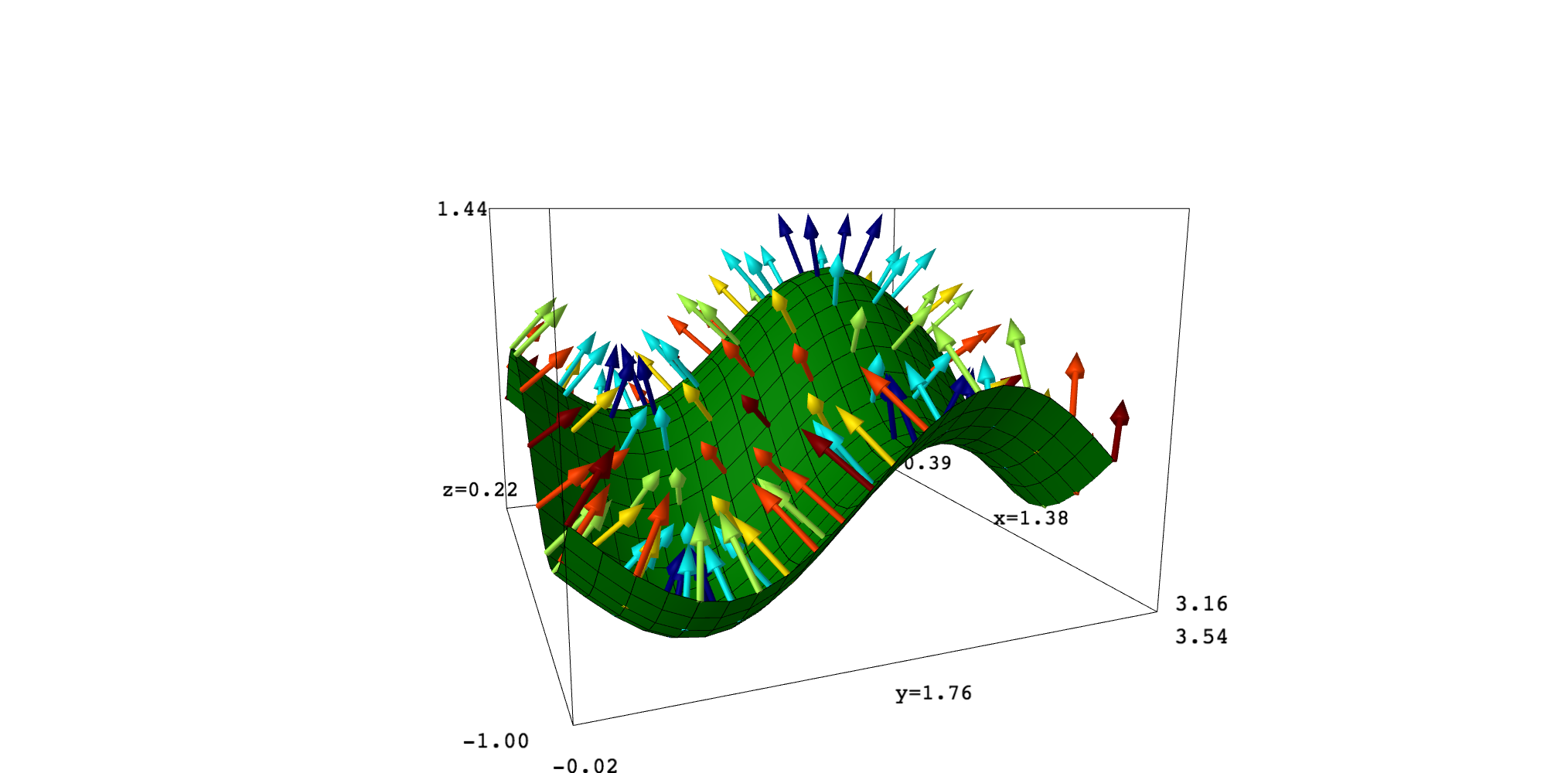I'm looking to plot the surface gradient vector field

$$\left \langle \frac{f_x}{\|\nabla f\|}, \frac{f_y}{\|\nabla f\|}, \|\nabla f\| \right \rangle$$

but only at points on the the surface $(x,y,f(x,y)$.

plotvectorfield3d will plot this field everywhere in 3D. I want to restrict this to the surface only.

Right now, I can plot these vectors individually by iterating along the surface, but I am wondering if someone has already tackled this more efficiently, perhaps with parameters on how to iterate.

Further, it would be nice to generalize this to directional derivatives for any unit vector $\mathbf{u} = \left \langle a, b \right \rangle$.

edit retag close merge delete

Sort by » oldest newest most votedI think that SageMath does not have a function behaving as you want, but you can define your own. In fact, it seems that this is what you are doing. On my side, I have ended with the following code which perhaps may be useful. It can be seem, as well as the examples, in this SageMath Cell. The image at the end corresponds to the second example.

def gradient_plot(fun, range_x, range_y, plot_points=10, color="red", radius=1/50, scale=1):
r"""
Plot gradient vectors of a bivariate function on points of its graph.
Arguments:
- fun: a symbolic expression corresponding to the function
- range_x and range_y: tuples of the form (var, min_value, max_value); the variables of
fun should be the first elements of these tuples
Options:
- plot_points: number of points in each direction; it can be a number or a list or a
tuple
- color: a specific color for all vectors or "gradient"; in this case, vectors are
colored depending on their norms
- scale: a factor to increase or decrease the length of the arrows

Example:
f(u,v) = u^2-v^2
g = gradient_plot(f(u,v), (u,-1,1), (v,-1,1), scale=0.5)
s = plot3d(f(u,v), (u,-1,1), (v,-1,1), mesh=True)
show(g+s)

Another example:
var("x,y")
fun = cos(x+y)*sin(x-y)
x1, x2, y1, y2 = 0, pi, 0, pi
surface = plot3d(fun, (x,x1,x2), (y,y1,y2), mesh=True, color="green", plot_points=21)
"""

# Process arguments
# -----------------
# fun

# ranges
var_x, xmin, xmax = range_x
var_y, ymin, ymax = range_y

# plot_points
if isinstance(plot_points, (list, tuple)):
nx, ny = plot_points
else:
nx = ny = plot_points

# color
color = "red"
else:

# Compute gradient vectors at surface points and obtain the
# corresponding arrows to be plotted
# Gradient norms are also stored
norms = []
arrows = []
dx, dy = (xmax-xmin)/(nx-1), (ymax-ymin)/(ny-1)
iter_x = [xmin,xmin+dx,..,xmax]
iter_y = [ymin,ymin+dy,..,ymax]
for xx in iter_x:
for yy in iter_y:
dic = {var_x: xx, var_y: yy}
p = [xx, yy, fun(dic)]
v = vector([-fx(dic), -fy(dic), 1])
nv = norm(v).n()
norms.append(nv)
v = scale*v/nv

# If required, modify arrow colors depending on the gradient norm.
# Colors range from dark blue (small norm) to dark red (big norm)
nvmin, nvmax = min(norms), max(norms)
if nvmin==nvmax: nvmin = 0
for i in range(len(arrows)):
icol = (norms[i]-nvmin)/(nvmax-nvmin)
vcol = colormaps.jet(icol)[:-1]
arrows[i].set_texture(color=vcol)
# End
return sum(arrows)Edit. The line fx, fy = fun.gradient() fails if fun is a constant or only contains one variable. So it is better to replace

fx, fy = fun.gradient()
var_x, xmin, xmax = range_x
var_y, ymin, ymax = range_y


by

var_x, xmin, xmax = range_x
var_y, ymin, ymax = range_y
fx, fy = fun.diff(var_x), fun.diff(var_y)

more

Thank you for this! What a wonderful template. I was looking to do vectors tangent to the surface in the direction of the gradient -- I modified your code slightly https://sagecell.sagemath.org/?q=tzrxaj (here) and it looks beautiful!

v = vector([fx(dic), fy(dic), ((fx(dic))^2+(fy(dic))^2)])

and then changed it to negative gradient descent (to simulate gravity along the surface)

v = scale*v/nv*-1

Greatly appreciated. I have also playing with embedding https://sagecell.sagemath.org/?q=rxmfpk (curves in 3D onto a surface) -- giving the curves some "thickness" is an interesting exercise, working on automating it, along with generating vector fields on it as above.

I've seen your tweet and the associated SageMath cell. Very nice picture!